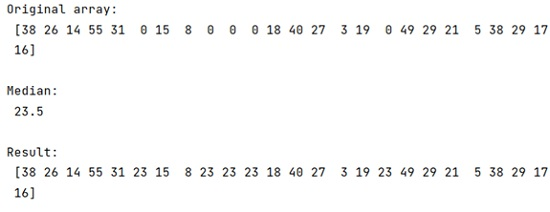# Replace Zeros with Median Value in NumPy Array

In this tutorial, we will learn how to replace zeros in NumPy array with the median value? By Pranit Sharma Last updated : April 22, 2023

## Problem Statement

Suppose that we are given a numpy array with some numerical values that include zeroes too but we need to replace the zeroes with the median of the array.

## What is median of a NumPy array?

The median of an array can be defined as the middle value of the array when the array is sorted and the length of the array is odd. In the case of an even number of elements, the median is the average of the middle two elements.

## How to calculate median of a NumPy array?

To calculate the median of the NumPy array, we can simply use numpy.median() method which calculates the median along the specified axis and the median of the array elements.

### Syntax:

```numpy.median(a, axis=None, out=None, overwrite_input=False, keepdims=False)
```

## How to replace zeros with median value in NumPy array?

To replace zeros with median value, you have to compute the median value first by using the numpy.median() method and extract the indices of zeros then assigns the median value to them using the following code snippet,

```ar[arr==0] = median_value
```

Let us understand with the help of an example,

## Python program to replace zeros with median value in NumPy array

```# Import numpy
import numpy as np

# Creating an array
arr = np.array([38,26,14,55,31,0,15,8,0,0,0,18,40,27,3,19,0,49,29,21,5,38,29,17,16])

# Display original array
print("Original array:\n",arr,"\n")

# Calculate median
med = np.median(arr[arr > 0])

# Print median
print("Median:\n",med,"\n")

# Replace zeros with median value
arr[arr==0] = med

# Print array after replacing zeros
# with median value
print("Result:\n",arr)
```

### Output